# Charting

## Matplotlib

### Introduction

matplotlib is the most popular 2d-charting library for python. It allows you to easily create histograms, scatter plots, and various other charts. In addition, pandas is integrated with matplotlib, so you can seamlessly move between data manipulation and data visualization. This makes matplotlib great for quickly producing a chart to visualize your data.

### Import Libraries

Follow these steps to import the libraries that you need:

1. Import the matplotlib, mplfinance, and numpy libraries.
2. import matplotlib.pyplot as plt
import mplfinance
import numpy as np
3. Import, and then call, the register_matplotlib_converters method.
4. from pandas.plotting import register_matplotlib_converters
register_matplotlib_converters()

### Get Historical Data

Get some historical market data to produce the plots. For example, to get data for a bank sector ETF and some banking companies over 2021, run:

qb = QuantBook()
tickers = ["XLF",   # Financial Select Sector SPDR Fund
"COF",   # Capital One Financial Corporation
"GS",    # Goldman Sachs Group, Inc.
"JPM",   # J P Morgan Chase & Co
"WFC"]   # Wells Fargo & Company
symbols = [qb.AddEquity(ticker, Resolution.Daily).Symbol for ticker in tickers]
history = qb.History(symbols, datetime(2021, 1, 1), datetime(2022, 1, 1))

### Create Candlestick Chart

You must import the plotting libraries and get some historical data to create candlestick charts.

In this example, we'll create a candlestick chart that shows the open, high, low, and close prices of one of the banking securities. Follow these steps to create the candlestick chart:

1. Select a Symbol.
2. symbol = symbols
3. Slice the history DataFrame with the symbol.
4. data = history.loc[symbol]
5. Rename the columns.
6. data.columns = ['Close', 'High', 'Low', 'Open', 'Volume']
7. Call the plot method with the data, chart type, style, title, y-axis label, and figure size.
8. mplfinance.plot(data,
type='candle',
style='charles',
title=f'{symbol.Value} OHLC',
ylabel='Price (\$)',
figratio=(15, 10))

The Jupyter Notebook displays the candlestick chart.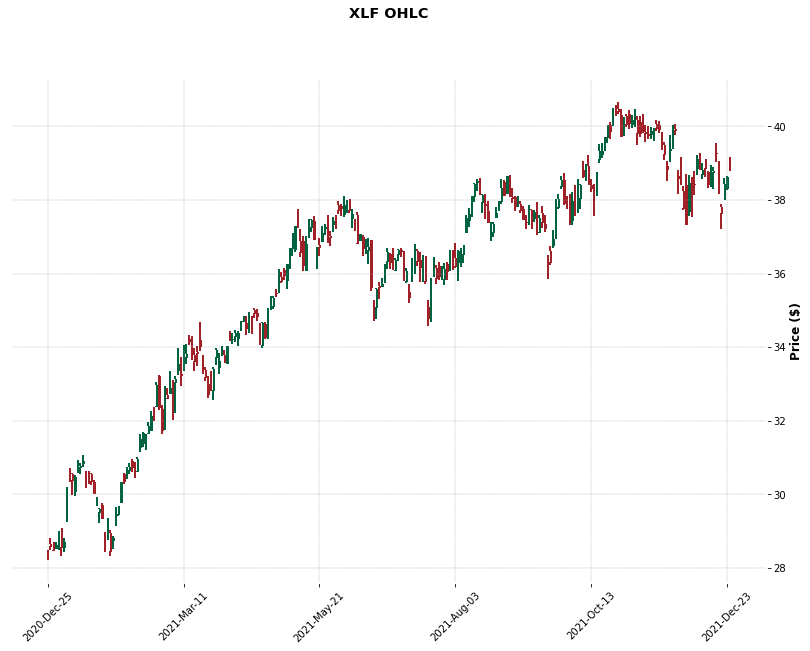### Create Line Plot

You must import the plotting libraries and get some historical data to create line charts.

In this example, you create a line chart that shows the closing price for one of the banking securities. Follow these steps to create the line chart:

1. Select a Symbol.
2. symbol = symbols
3. Slice the history DataFrame with symbol and then select the close column.
4. data = history.loc[symbol]['close']
5. Call the plot method with a title and figure size.
6. data.plot(title=f"{symbol} Close Price", figsize=(15, 10));

The Jupyter Notebook displays the line plot.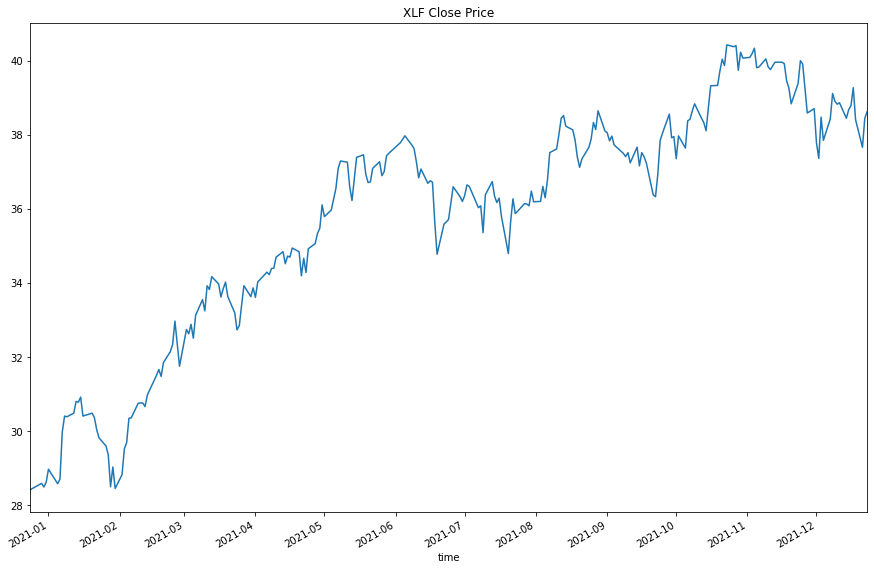### Create Scatter Plot

You must import the plotting libraries and get some historical data to create scatter plots.

In this example, you create a scatter plot that shows the relationship between the daily returns of two banking securities. Follow these steps to create the scatter plot:

1. Select the 2 Symbols.
2. For example, to select the Symbols of the first 2 bank stocks, run:

symbol1 = symbols
symbol2 = symbols
3. Slice the history DataFrame with each Symbol and then select the close column.
4. close_price1 = history.loc[symbol1]['close']
close_price2 = history.loc[symbol2]['close']
5. Call the pct_change and dropna methods on each Series.
6. daily_returns1 = close_price1.pct_change().dropna()
daily_returns2 = close_price2.pct_change().dropna()
7. Call the polyfit method with the daily_returns1, daily_returns2, and a degree.
8. m, b = np.polyfit(daily_returns1, daily_returns2, deg=1)

This method call returns the slope and intercept of the ordinary least squares regression line.

9. Call the linspace method with the minimum and maximum values on the x-axis.
10. x = np.linspace(daily_returns1.min(), daily_returns1.max())
11. Calculate the y-axis coordinates of the regression line.
12. y = m*x + b
13. Call the plot method with the coordinates and color of the regression line.
14. plt.plot(x, y, color='red')
15. In the same cell that you called the plot method, call the scatter method with the 2 daily return series.
16. plt.scatter(daily_returns1, daily_returns2)
17. In the same cell that you called the scatter method, call the title, xlabel, and ylabel methods with a title and axis labels.
18. plt.title(f'{symbol1} vs {symbol2} daily returns Scatter Plot')
plt.xlabel(symbol1.Value)
plt.ylabel(symbol2.Value);

The Jupyter Notebook displays the scatter plot.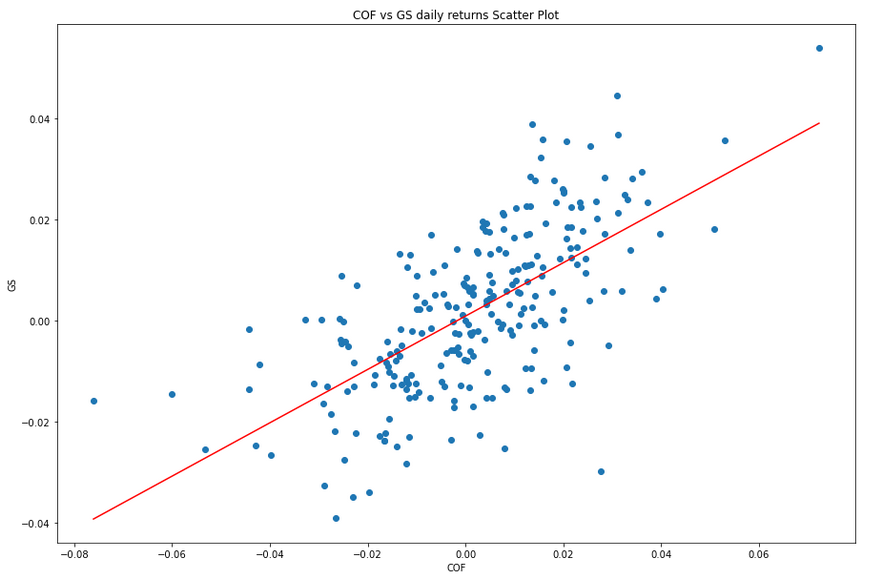### Create Histogram

You must import the plotting libraries and get some historical data to create histograms.

In this example, you create a histogram that shows the distribution of the daily percent returns of the bank sector ETF. In addition to the bins in the histogram, you overlay a normal distribution curve for comparison. Follow these steps to create the histogram:

1. Select the Symbol.
2. symbol = symbols
3. Slice the history DataFrame with the symbol and then select the close column.
4. close_prices = history.loc[symbol]['close']
5. Call the pct_change method and then call the dropna method.
6. daily_returns = close_prices.pct_change().dropna()
7. Call the mean and std methods.
8. mean = daily_returns.mean()
std = daily_returns.std()
9. Call the linspace method with the lower limit, upper limit, and number data points for the x-axis of the normal distribution curve.
10. x = np.linspace(-3*std, 3*std, 1000)
11. Calculate the y-axis values of the normal distribution curve.
12. pdf = 1/(std * np.sqrt(2*np.pi)) * np.exp(-(x-mean)**2 / (2*std**2))
13. Call the plot method with the data for the normal distribution curve.
14. plt.plot(x, pdf, label="Normal Distribution")
15. In the same cell that you called the plot method, call the hist method with the daily return data and the number of bins.
16. plt.hist(daily_returns, bins=20)
17. In the same cell that you called the hist method, call the title, xlabel, and ylabel methods with a title and the axis labels.
18. plt.title(f'{symbol} Return Distribution')
plt.xlabel('Daily Return')
plt.ylabel('Count');

The Jupyter Notebook displays the histogram.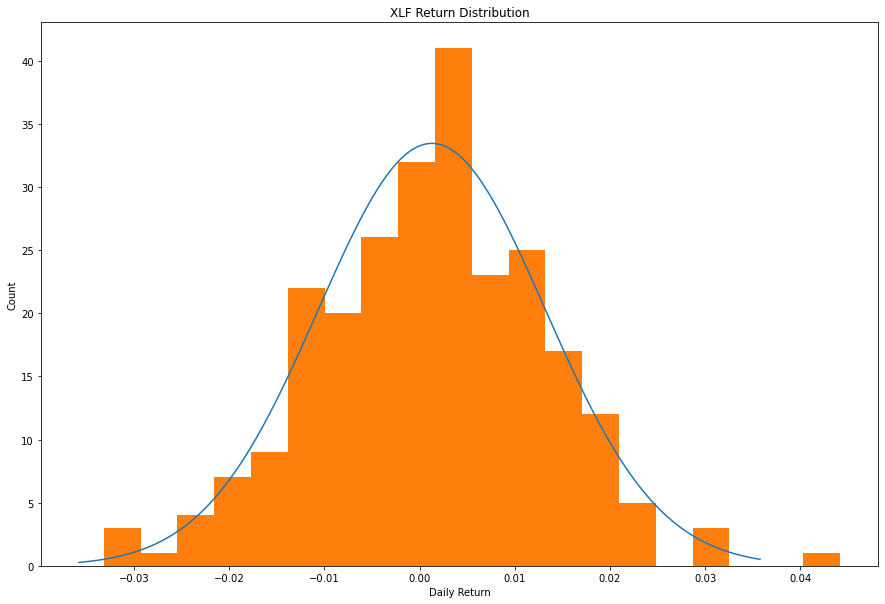### Create Bar Chart

You must import the plotting libraries and get some historical data to create bar charts.

In this example, you create a bar chart that shows the average daily percent return of the banking securities. Follow these steps to create the bar chart:

1. Select the close column and then call the unstack method.
2. close_prices = history['close'].unstack(level=0)
3. Call the pct_change method and then multiply by 100.
4. daily_returns = close_prices.pct_change() * 100
5. Call the mean method.
6. avg_daily_returns = daily_returns.mean()
7. Call the figure method with a figure size.
8. plt.figure(figsize=(15, 10))
9. Call the bar method with the x-axis and y-axis values.
10. plt.bar(avg_daily_returns.index, avg_daily_returns)
11. In the same cell that you called the bar method, call the title, xlabel, and ylabel methods with a title and the axis labels.
12. plt.title('Banking Stocks Average Daily % Returns')
plt.xlabel('Tickers')
plt.ylabel('%');

The Jupyter Notebook displays the bar chart.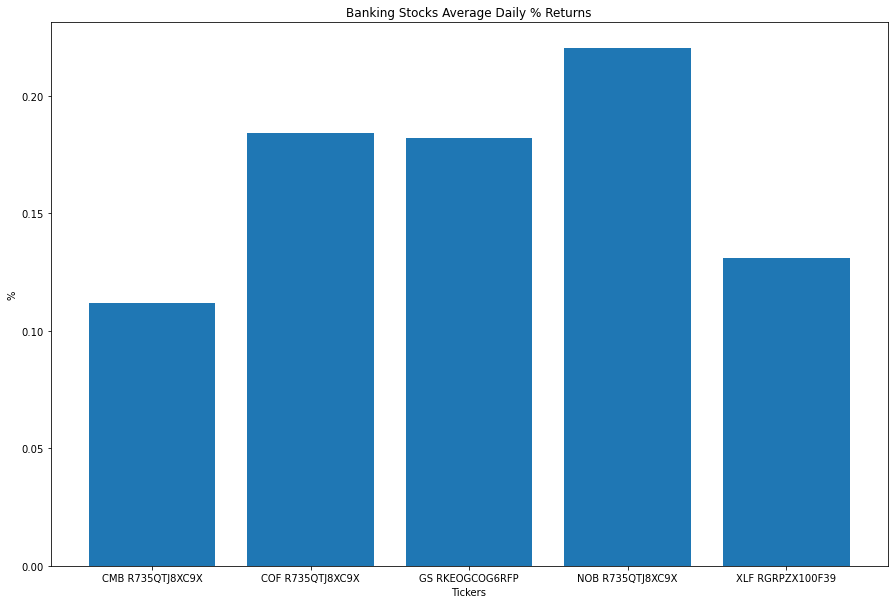### Create Heat Map

You must import the plotting libraries and get some historical data to create heat maps.

In this example, you create a heat map that shows the correlation between the daily returns of the banking securities. Follow these steps to create the heat map:

1. Select the close column and then call the unstack method.
2. close_prices = history['close'].unstack(level=0)
3. Call the pct_change method.
4. daily_returns = close_prices.pct_change()
5. Call the corr method.
6. corr_matrix = daily_returns.corr()
7. Call the imshow method with the correlation matrix, a color map, and an interpolation method.
8. plt.imshow(corr_matrix, cmap='hot', interpolation='nearest')
9. In the same cell that you called the imshow method, call the title, xticks, and yticks, methods with a title and the axis tick labels.
10. plt.title('Banking Stocks and Bank Sector ETF Correlation Heat Map')
plt.xticks(np.arange(len(tickers)), labels=tickers)
plt.yticks(np.arange(len(tickers)), labels=tickers)
11. In the same cell that you called the imshow method, call the colorbar method.
12. plt.colorbar();

The Jupyter Notebook displays the heat map.### Create Pie Chart

You must import the plotting libraries and get some historical data to create pie charts.

In this example, you create a pie chart that shows the weights of the banking securities in a portfolio if you allocate to them based on their inverse volatility. Follow these steps to create the pie chart:

1. Select the close column and then call the unstack method.
2. close_prices = history['close'].unstack(level=0)
3. Call the pct_change method.
4. daily_returns = close_prices.pct_change()
5. Call the var method and then take the inverse.
6. inverse_variance = 1 / daily_returns.var()
7. Call the pie method with the inverse_variance Series, the plot labels, and a display format.
8. plt.pie(inverse_variance, labels=inverse_variance.index, autopct='%1.1f%%')
9. In the cell that you called the pie method, call the title method with a title.
10. plt.title('Banking Stocks and Bank Sector ETF Allocation');

The Jupyter Notebook displays the pie chart.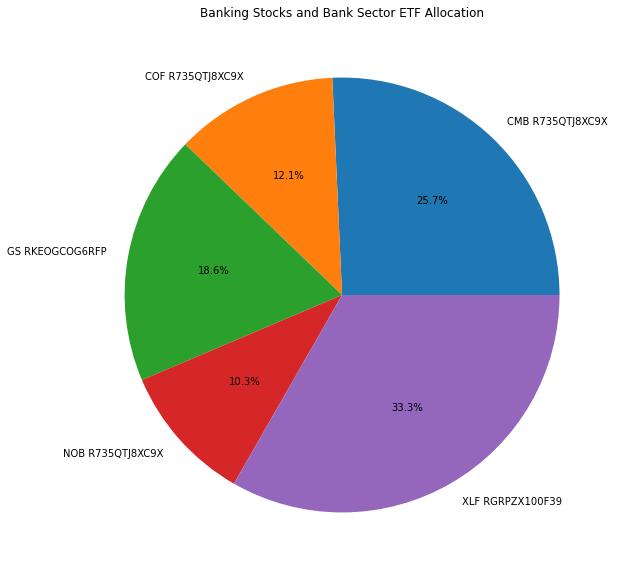You can also see our Videos. You can also get in touch with us via Discord.